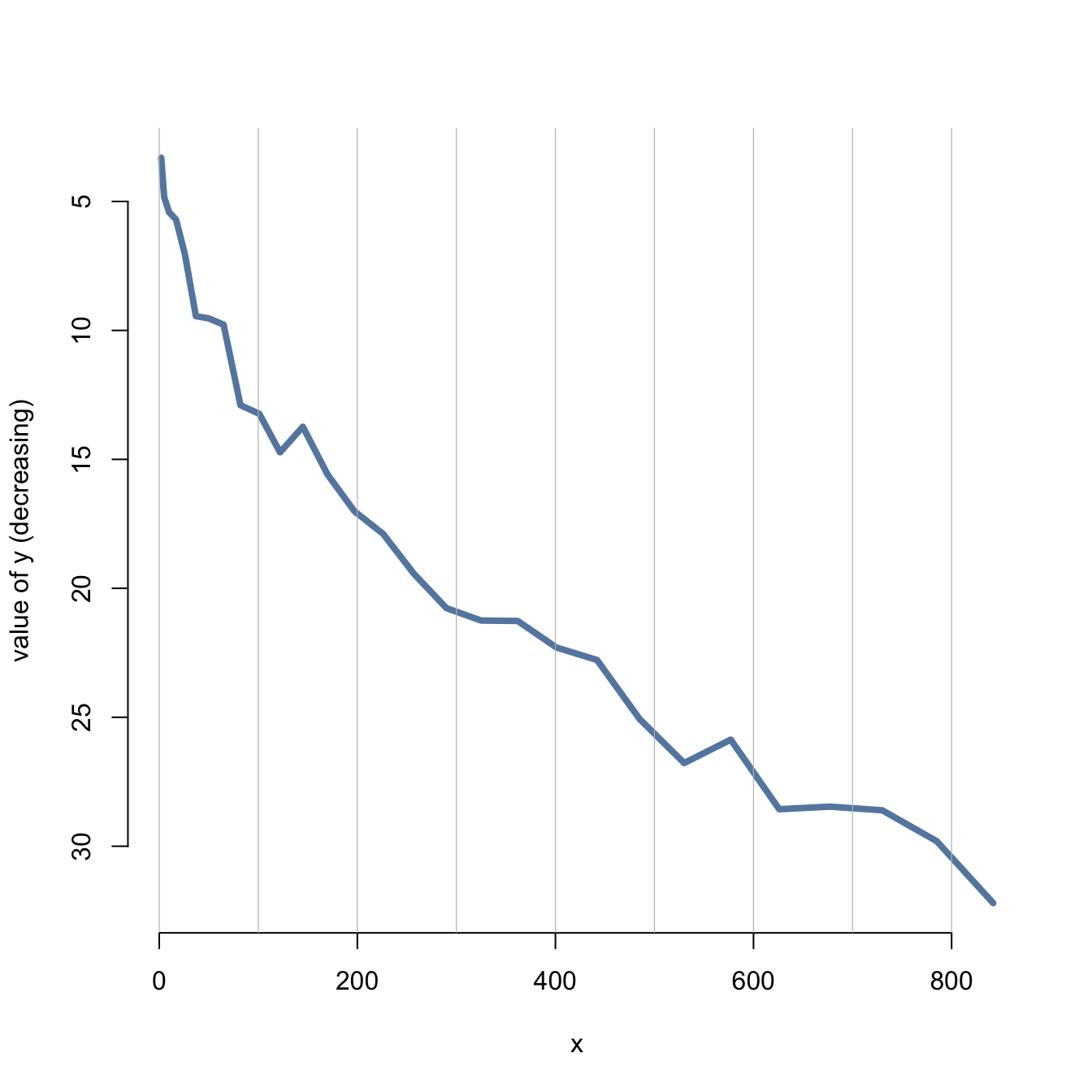# Reversed Y axis in base R

This post explains how to reverse the Y axis on a chart with `R`. It uses a line chart as example and provides the reproducible code.

It is a common need in dataviz to flip the Y axis upside down. In base R this is pretty easy to do: you just have to reverse the values of the `ylim` argument.

This can be done using this statement: `rev(range(y))`

Note: be carefull, do not build a counter-iintuitive chart``````# Create data
x <- seq(1,29)^2+runif(29,0.98)
y <- abs(seq(1,29)+4*runif(29,0.4))

# Make the plotwith ylim in reverse
plot(y~x , ylim = rev(range(y)) ,
lwd=4 , type="l" , bty="n" , ylab="value of y (decreasing)" , col=rgb(0.2,0.4,0.6,0.8) )